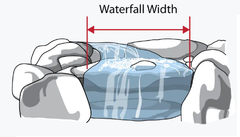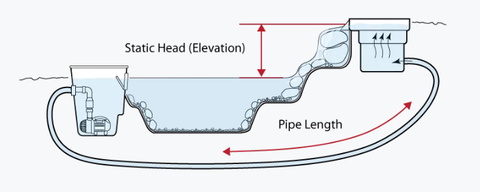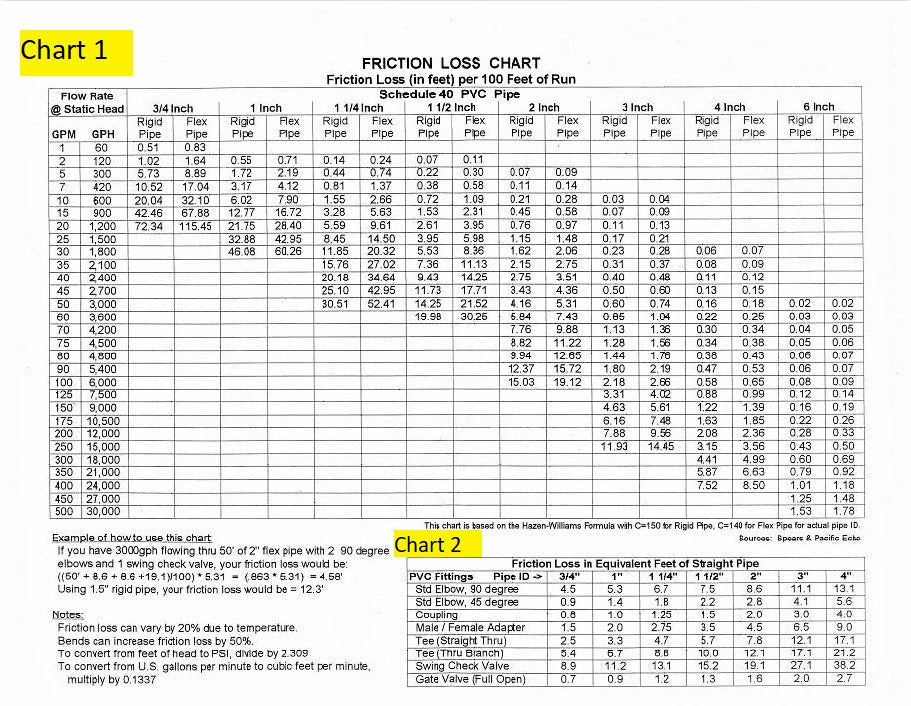# Pond Pump Sizing Reference Calculators

Step 1.  Pond Volume

In other words, how many gallons of water are in your pond? Gallons are figured by multiplying the average length (ft) x average width (Ft) x average depth (ft) x 7.5. There are differing opinions pertaining to recirculation values, but a good rule of thumb is to recirculate the entire volume of water at least once an hour.  Use this calculator to determine your pond volume.  Enter your length, width, and average depth.  The pond volume will be calculated at 80% of the total volume, which accounts for rocks in the pond.

Step 2.  Waterfall Width

It is recommended between 100 - 200 gallons per hour (GPH) for every inch of waterfall width.  On the lower flow rate (100 GPH) will give you a slower stream vs the higher flow rate (200 GPH) will give you a faster stream over your waterfall.  Aquascape recommends 125 GPH for every inch of waterfall.  Use this calculator to help you calculate your low and high flow rates.Choose the higher of the two numbers calculated above in steps 1 and 2 (Pond Volume and Waterfall Flow Rate) to determine your "Recommended Flow Rate".  From here, you need to calculate your Static Head.Elevation (Static Head):  Height difference from water level in the feature to the top of the waterfall.

Pipe Length:  Total length of pipe (10 feet of pipe = 1 foot of head height)

Fittings Used (For simple ponds, this can be ignored): 90-degree fittings (equate to 2 feet of head height) and all other fittings (1 foot of head height) contribute to head losses.   However, on larger applications you also need to factor in friction loss from the pipe as well as each fitting to get the Total Dynamic Head (TDH), shown in Step 5.

Use the calculator below to calculate Static Head.

Take your Recommended Flow Rate and your Head Height, both found in Step 3, and use this to determine the "Best Operating Zone" on the Pump Flow Chart. Below is an example

of a Pump Flow Chart. As an example, if you're Recommended Flow Rate was 3,500 GPH with 15' of Head Height, a great choice would be the 5-PL Pump because it is within the "Best

Operating Zone" shown in green with an "Application Head Height" in the range of 10' to 18'.Step 5. Calculate Total Dynamic Head for Larger Applications

Total Dynamic Head is the Static Head, found in Step 3 + the friction loss created by the pipe and the fittings.  In most small ponds with short pipe runs friction loss is not typically a problem.  However, in a with long streams or high flow rates, friction loss can have a big impact on the performance of the pump.

Use our calculator below to find your Total Dynamic Head.   Friction Loss numbers are available on Chart 1 & Chart 2 below and are based on your diameter of pipe and whether it is flexible PVC or rigid PVC.

Here's an example on obtaining friction loss numbers: We are pumping 4200 GPH through 100' of 2" flex pipe.  We are also using 2 - 2" 90-degree elbows and 1 - 2" check valve.

Friction Loss for the 2" Flex Pipe:  Using Chart 1, the pipe friction loss for the 2" pipe is 9.98.  Follow the 4,200 GPH in the second column from the left over to the 2-inch Flex Pipe column.

Friction Loss for the 2" 90-Degree Elbows:  Using Chart 2, the pipe friction loss for the 2" - 90-Degree Elbows is 8.6 for EACH elbow.  Follow the "Std Elbow, 90 Degree" in the left column over to the "2" " column.

Friction Loss for the 2" Check Valve:  Using Chart 2, the pipe friction loss for the 2" - Check Valve is 19.1.  Follow the "Swing Check Valve" in the left column over to the "2" " column.

These friction loss numbers shown in the above example can be added to the Total Dynamic Head Calculator below.